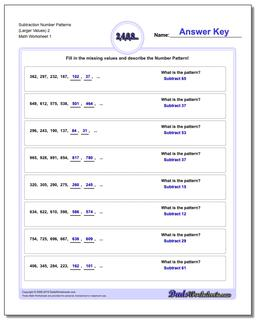# Math Worksheets: Number Patterns: Number Patterns: Subtraction Number Patterns (Larger Values) 2## Subtraction Number Patterns (Larger Values) 2

PropertyValue
DescriptionSubtraction Number Patterns (Larger Values) 2: Number pattern problems using only subtraction operations.
Resource TypeWorksheet